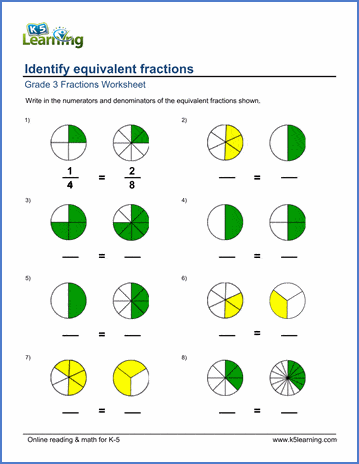# Fractions Worksheets

Our fraction worksheets start with the introduction of the concepts of "equal parts", "parts of a whole" and "fractions of a group or set"; and proceed to operations on fractions and mixed numbers.

Fraction worksheets

Fractions to decimals

Fraction multiplication and division

Converting fractions, equivalent fractions, simplifying fractions

Fraction to / from decimals

Fraction multiplication and division worksheets

Converting fractions, equivalent fractions, simplifying fractions

Fraction to / from decimals

Topics include:

• Identifying "equal parts"
• Dividing shapes into "equal parts"
• Parts of a whole
• Fractions in words
• Coloring shapes to make fractions
• Writing fractions
• Fractions of a group or set
• Word problems: write the fraction from the story

• Equal parts
• Numerators and denominators of a fraction
• Writing fractions from a numerator and denominator
• Reading fractions and matching to their words
• Writing fractions in words
• Identifying common fractions (matching, coloring, etc)
• Fractions as part of a set or group (identifying, writing, coloring, etc)
• Using fractions to describe a set
• Comparing fractions with pie charts (parts of whole, same denominator)
• Comparing fractions with pie charts (same numerator, different denominators)
• Comparing fractions with pictures (parts of sets)
• Comparing fractions with block diagrams
• Understanding fractions word problems
• Writing and comparing fractions word problems

• Identifying fractions
• Writing fractions
• Fractional part of a set
• Identifying equivalent fractions
• Equivalent fractions - missing numerators, denominators
• 3 Equivalent fractions
• Comparing fractions with pie charts (same denominator)
• Comparing proper fractions with pie charts
• Comparing proper or improper fractions with pie charts
• Compare mixed numbers with pie charts
• Comparing fractions (like, unlike denominators)
• Compare improper fractions, mixed numbers
• Simplifying fractions (proper, improper)
• Completing whole numbers
• Subtracting like fractions
• Subtracting a fraction from a whole number or mixed number
• Subtracting mixed numbers
• Converting fractions to / from mixed numbers
• Converting mixed numbers and fractions to / from decimals
• Fractions word problems

• Adding like fractions (denominators 2-12)
• Adding like fractions (all denominators)
• Adding fractions and mixed numbers (like denominators)
• Completing whole numbers
• Subtracting like fractions (denominators 2-12)
• Subtracting fractions from whole numbers, mixed numbers
• Subtracting mixed numbers from mixed numbers or whole numbers
• Converting fractions to / from mixed numbers
• Comparing proper fractions with pie charts
• Comparing improper fractions and mixed numbers with pie charts
• Comparing proper and improper fractions
• Ordering 3 fractions
• Identifying equivalent fractions (pie charts)
• Writing equivalent fractions (pie charts)
• Equivalent fractions with missing numerators or denominators
• Fractions word problems

## Grade 4 fractions to decimals worksheets

• Convert decimals to fractions (tenths, hundredths)
• Convert decimals to mixed numbers (tenths, hundredths)
• Convert fractions to decimals (denominator of 10 or 100)
• Convert mixed numbers to decimals (denominator of 10 or 100)

• Adding like fractions (denominators 2-25)
• Adding mixed numbers and / or fractions (like denominators)
• Completing whole numbers
• Adding unlike fractions & mixed numbers
• Subtracting like fractions
• Subtracting fractions from whole numbers and mixed numbers (same denominators)
• Subtracting mixed numbers with missing subtrahend or minuend)
• Subtracting unlike fractions
• Subtracting mixed numbers (unlike denominators)
• Word problems on adding and subtracting fractions

## Grade 5 fraction multiplication and division worksheets

• Multiply fractions by whole numbers
• Multiply fractions by fractions
• Multiply improper fractions
• Multiply fractions by mixed numbers
• Multiply mixed numbers by mixed numbers
• Missing factor questions
• Divide whole numbers by fractions (answers are whole numbers)
• Divide a fraction by a whole number and vice versa
• Divide mixed numbers by fractions
• Divide fractions by fractions
• Mixed numbers divided by mixed numbers
• Word problems on multiplying and dividing fractions
• Mixed operations with fractions word problems

## Grade 5 converting, simplifying & equivalent fractions

• Converting improper fractions to / from mixed numbers
• Simplifying proper fractions
• Simplifying proper and improper fractions
• Equivalent fractions (2 fractions)
• Equivalent fractions (3 fractions)

## Grade 5 fraction to / from decimals worksheets

• Convert decimals to fractions (tenths, hundredths), no simplification
• Convert decimals to fractions (tenths, hundredths), with simplification
• Convert decimals to mixed numbers
• Convert fractions to decimals (denominators of 10 or 100)
• Convert mixed numbers to decimals (denominators of 10 or 100)
• Convert mixed numbers to decimals (denominators of 10, 100 or 1000)
• Convert fractions to decimals (common denominators of 2, 4, 5, ...)
• Convert mixed numbers to decimals (common denominators of 2, 4, 5, ...)
• Convert fractions to decimals, some with repeating decimals

• Adding  fractions and mixed numbers
• Adding mixed numbers (unlike denominators)
• Subtract unlike fractions
• Subtract mixed numbers (unlike denominators)

## Grade 6 fraction multiplication and division worksheets

• Fractions multiplied by whole numbers
• Fractions multiplied by fractions
• Mixed numbers multiplied by fractions
• Mixed numbers multiplied by mixed numbers
• Whole numbers divided by fractions
• Fractions divided by fractions
• Mixed numbers divided by mixed nuymbers
• Mixed multiplication or division practice

## Grade 6 converting, simplifying and equivalent fractions worksheets

• Convert improper fractions to / from mixed numbers
• Simplify proper fractions
• Simplify proper and improper fractions
• Equivalent fractions (2 fractions)
• Equivalent fractions (3 fractions)
• Equivalent fractions (4 fractions)

## Grade 6 fraction to / from decimals worksheets

• Convert decimals to fractions, with simplification
• Convert decimals to mixed numbers, with simplification
• Convert fractions to decimals (denominators are 10 or 100)
• Convert fractions to decimals (various denominators)
• Convert mixed numbers to decimals (various denominators)

## Related topics

Decimals worksheets

Word problem worksheetsSample Fractions Worksheet

What is K5?

K5 Learning offers free worksheets, flashcards and inexpensive workbooks for kids in kindergarten to grade 5. Become a member to access additional content and skip ads.